Practice Questions with Solutions: Operations on Large Numbers

# Practice Questions with Solutions: Operations on Large Numbers - Notes | Study Mathematics for Class 5 - Class 5

 1 Crore+ students have signed up on EduRev. Have you?

Question 1: In a factory, there are 519 workers. The salary of each worker is ₹ 1,895 per month. What is the total expenditure on the salary of the workers per month?

Salary of 1 worker = ₹ 1,895 per month

Number of workers = 519

Total expenditure on salary of workers per month = ₹1,895 × 519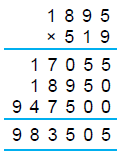Hence, the expenditure on the salary of the workers is ₹ 9,83,505 per month.

Question 2: Estimate the product of 563 and 84.

563 → 600 (Rounding off to hundred’s place)
84 → 80 (Rounding off to ten’s place)
563 × 84 → 600 × 80 i.e., 48,000.
Estimated product is 48,000.
To find the estimated quotient, the dividend and the divisor are rounded off to their greatest place and their division gives the estimated quotient.

Question 3: Add 4,34,20,911; 46,35,194 and 5,61,321.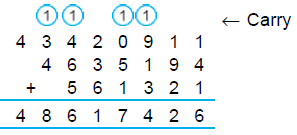Hence, 4,34,20,911 + 46,35,194 + 5,61,321 = 4,86,17,426

Question 4: Find the difference between 5,41,72,384 and 60,00,32,107.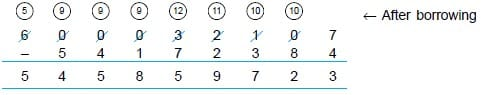Thus, the difference between 5,41,72,384 and 60,00,32,107 is 54,58,59,723

Question 5: In 2012, a factory produced 50,39,854 bulbs and in 2013, it produced 47,80,362 bulbs. Find the total number of bulbs produced by the factory in these two years.

Number of bulbs produced by the factory in 2012 = 50,39,854
Number of bulbs produced by the factory in 2013 = 47,80,362
Total number of bulbs produced = 50,39,854 + 47,80,362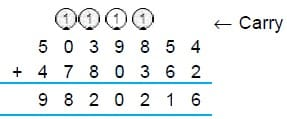Question 6:

(a) Estimate the difference 3,587 – 1,329
(i) to the nearest tens (ii) to the nearest hundreds
(b) Which of them is closer to the actual difference?

(a)
(i) Rounding off to nearest 10, we have
3,587 → 3,590      [7 > 5 ⇒ 7 → 10 and 87 → 90] and 1,329 → 1,330    [9 > 5 ⇒ 9 → 10 and 29 → 30]
Thus, 3,587 – 1,329 → 3,590 – 1,330 = 2,260

(ii) Rounding off to nearest 100, we have

3,587→3,600 [8 > 5 ⇒ 87 → 100 or 587 → 600] and 1329 → 1,300 [2 < 5 ⇒ 29 → 00 or 329 → 300]

3,587 – 1,329 → 3,600 – 1,300 = 2,300

(b) Actual difference = 3,587 – 1,329 = 2,258.

The first approximation (to the nearest 10) 2,260 is closer to the actual difference

which is 2,258.

Question 7: 50,02,519 students appeared for the Board Examination. Out of them, 32,50,874 were boys. How many girls appeared for the examination?

Total number of students appeared = 50,02,519

Number of boys = 32,50,874

Number of girls = 50,02,519 – 32,50,874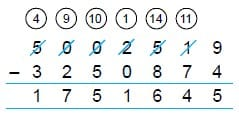Hence, 17,51,645 girls appeared for the examination.

Question 8: 155 matchboxes are packed in a carton. How many cartons will be needed to pack 3,16,665 matchboxes?

Number of matchboxes packed in 1 carton = 155
Total number of matchboxes to be packed = 3,16,665
Number of cartons needed = 3,16,665 ÷  155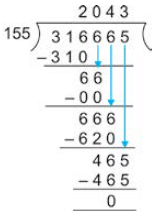Hence, 2,043 cartons are needed.

Question 9: A packet contains 1,215 nails. How many nails are there in 2,715 packets?

Nails in 1 packet = 1,215

Number of packets = 2,715

Total number of nails = 1,215 × 2,715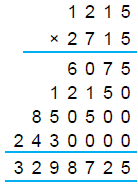Hence, the required number of nails are 32,98,725.

Question 10: Divide 4,63,806 by 42.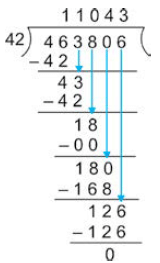Thus, 4,63,806 ÷ 42 = 11,043.

Question 11: Estimate the sum 3,660 + 7,948 to the nearest tens and verify its closeness to the actual sum.

On estimating to nearest tens, we have :
3,660 → 3,660    [0 < 5 ⇒ 0 → 0]
7,948 → 7,950   [8 > 5 ⇒ 8 → 10 or 48 → 50]

3,660 + 7,948 → 3,660 + 7,950 = 11,610.
Estimated sum = 11,610
Actual sum = 3,660 + 7,948 = 11,608

Question 12: Multiply 13,578 by 329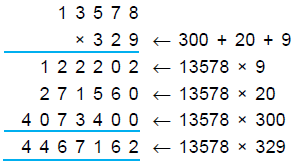Since, 329 = 300 + 20 + 9, we multiply 13,578 by 9, 20 and 300 respectively. Finally,
we add the results to get the required product.

Question 13: Add 25,13,071 and 43,76,82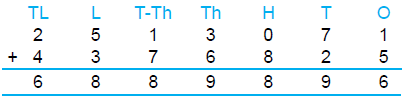Hence, 25,13,071 + 43,76,825 = 68,89,896.

Question 14: What must be added to 51,35,94,612 to get 85,39,26,140?

Sum of two numbers = 85,39,26,140

One number = 51,35,94,612
The other number = 85,39,26,140 – 51,35,94,612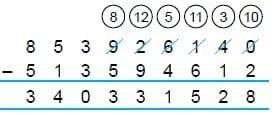Hence, the required number = 34,03,31,528.

Question 15: In a city, there are 2,35,01,898 men, 1,56,70,314 women and 51,29,417 children. What is the total population of the city?

Number of men = 2,35,01,898
Number of women = 1,56,70,314
Number of children = 51,29,417
So, total population = 2,35,01,898 + 1,56,70,314 + 51,29,417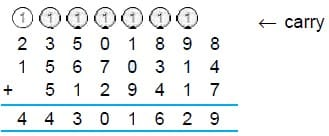Hence, the total population of the city is 4,43,01,629.

Question 16: The cost of 62 computers is ₹ 14,06,346. Find the cost of 1 computer.

Cost of 62 computers = ₹ 14,06,346.

Cost of 1 computer = ₹ 14,06,346 ÷  62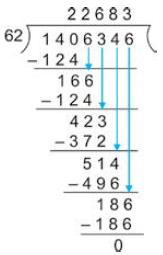Hence, the cost of 1 computer is ₹ 22,683.

Question 17: Subtract 4,32,70,156 from 8,46,93,587.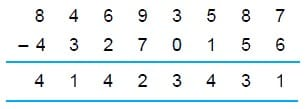Thus, 8,46,93,587 – 4,32,70,156 = 4,14,23,431.

Question 18: Estimate the quotient for 4,683 ÷ 172.

Here 4,683 is rounded to 5,000 (nearest 1,000)
172 is rounded to 200 (nearest 100)
5,000 ÷ 200 gives a quotient = 25
Estimated quotient = 25.

Question 19: Multiply 4,295 by 1,236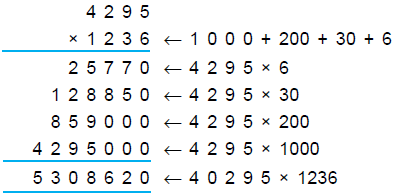Question 20: The population of a city is 8,05,03,624. There are 3,53,72,460 men and 3,00,04,738 women. The rest of them are children. Find the number of children in the city.

Number of men = 3,53,72,460
Number of women = 3,00,04,738
Total number of men and women in the city = 3,53,72,460 + 3,00,04,738.
= 6,53,77,198.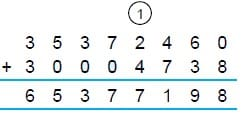Total population of the city = 8,05,03,624
Number of children in the city = 8,05,03,624 – 6,53,77,198
Hence, the number of children in the city is 1,51,26,426.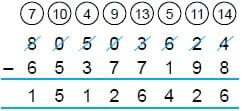Question 21: Estimate the above sum
(a) to the nearest 100
(b) to the nearest 1,000.
Which estimation is the closest to the actual sum?

(a) Rounding off to nearest 100, we have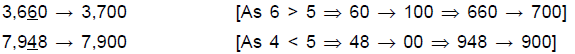Thus, 3,660 + 7,948 → 3,700 + 7,900

i.e., 3,660 + 7,948 → 3,700 + 7,900 = 11,600.

(b) Rounding off the same numbers to nearest 1,000, we have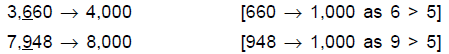3,660 + 7,948 → 4,000 + 8,000 = 12,000
Approximation to nearest 10 gives the sum as 11,610.
Approximation to nearest 100 gives the sum as 11,600.
Approximation to nearest 1,000 gives the sum as 12,000.

Out of the three sums 11,610, 11,600 and 12,000, we find that the first approximation ( to the nearest 10) is the closest to the actual sum, which is 11,608.

Question 22: Divide 59,41,025 by 139 and check the answer.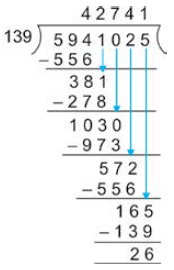Hence, quotient = 42,741, remainder = 26.
check : Dividend = quotient × divisor + remainder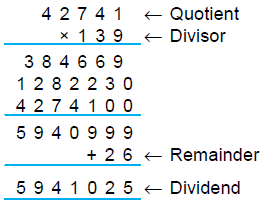Hence, the division is correct.

The document Practice Questions with Solutions: Operations on Large Numbers - Notes | Study Mathematics for Class 5 - Class 5 is a part of the Class 5 Course Mathematics for Class 5.
All you need of Class 5 at this link: Class 5

## Mathematics for Class 5

12 videos|55 docs|35 tests
 Use Code STAYHOME200 and get INR 200 additional OFF

## Mathematics for Class 5

12 videos|55 docs|35 tests

Track your progress, build streaks, highlight & save important lessons and more!

,

,

,

,

,

,

,

,

,

,

,

,

,

,

,

,

,

,

,

,

,

;